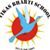Trending ▼   ResFinder# ICSE Class IX Prelims 2019 : Computer Science (Vikas Bharti School, Gorakhpur)

6 pages, 7 questions, 0 questions with responses, 0 total responses,00Vikas Bharti +Fave Message ProfileTimelineUploads
 Home > sanjeevvbs >   F Also featured on: School PageFormatting page ...

Vikas Bharti School Bhartipuram, Gorakhpur Mock Trials 2019 (Set - A) COMPUTER APPLICATIONS (Two Hours) Answers to this Paper must be written on the paper provided separately. You will not be allowed to write during the first 15 minutes. This time is to be spent in reading the question paper. The time given at the head of this Paper is the time allowed for writing the answers. This Paper is divided into two Sections. Attempt all questions from Section A and any four questions from Section B. The intended marks for questions or parts of questions are given in brackets [ ]. SECTION A (40 Marks) Attempt all questions Question 1: a) Why do we need a constructor as a class member?  b) Name the following:  i. A package that is invoked by default. ii. A key word, to use the classes defined in a packeage. c) What is the difference between length and length( ) function?  d) Give the prototype of the function Sum which receives a character (ch) and an integer (n) and returns true or false.  e) What is an infinite loop? Give one example of infinite loop statement.  Question 2: a) If int arr[ ]= {2,6,9,4,7,10,3,5,11}; what will be values of X and Y? X= arr.length; Y= (arr + arr *arr); b) Give the output of the following: a+= a++ + ++a + -- a + a--; when a =10; c) Give the output of the following: String S= Make in India ; String SS= Made in India ; i. System.out.prinln(S.compareTo(SS)); ii. System.out.prinln(S.substring(0,4)+SS.substrig(5)); d) Arrange the given primitive data types in an ascending order of their size. char, int, byte, double e) Name any two OOP s principles. f) Rewrite the following program segment using if..else statements: String grade = (mark>=90)? A :( mark>=80)? B : C ; g) Differentiate between switch statement and if..else statement. h) Write the java expression for: ut + 3/4ft2 + g3 i) What is the data type that the following library functions return?Formatting page ...Formatting page ...Formatting page ...Formatting page ...Formatting page ...